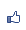Contents ...
udn網路城邦

2015/10/06 06:48ChiCheng Hsu

……

Where the Spring is Hsu ChiCheng

Where the warmth of golden sun is where the spring is
Where the caress of the breeze is where the spring is
Where the moist of the drizzle is where the spring is
Where the burst of the bud is where the spring is
Where the greenness of the leaves is where the spring is
Where the singing of the insects and the birds is where the spring is
Where the dance of the butterflies is where the spring is
Where the mew of the cats is where the spring is
……
As opinions vary
The best version is:
Where the laughter of the public is where the spring is

2015/10/6 World of Poets